# GED Math : Scientific Notation

## Example Questions

1 3 Next →

### Example Question #124 : Numbers

How will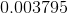be expressed in scientific notation?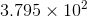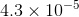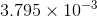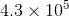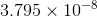Explanation:

The rules of scientific notation are simple. A number is being converted into a format where it's a decimal between 1 and just below 10 (9.9999999...) accompanied by an exponent. The exponent represents how many times to the decimal must move to the left or to the right to become the original number again. Scientific notation does not change the value of the number, but merely provide number in an easier to look at way. If the decimal must move to the right in order to achieve the original number, it will be represented by a positive exponent. If the decimal must move to the left, it will be represented by a negative exponent.

For this example,we first write how this number would be if it were in decimal form (between 1 and just shy of 10).

This would be :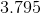Now we must count how many times we had to move the decimal over to achieve this decimal. (Remember where the decimal is in the original number.) We can see that the decimal had to movetimes. With the decimal placed between theand, we see that if we wanted to go back to the original number (), we would have to move the decimal to the left. This means a negative exponent. Therefore, the answer would be:### Example Question #21 : Scientific Notation

Express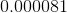in scientific notation.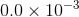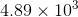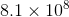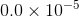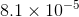Explanation:

The rules of scientific notation are simple. A number is being converted into a format where it's a decimal between 1 and just below 10 (9.9999999...) accompanied by an exponent. The exponent represents how many times to the decimal must move to the left or to the right to become the original number again. Scientific notation does not change the value of the number, but merely provide number in an easier to look at way. If the decimal must move to the right in order to achieve the original number, it will be represented by a positive exponent. If the decimal must move to the left, it will be represented by a negative exponent.

For this example,we first write how this number would be if it were in decimal form (between 1 and just shy of 10).

This would be :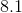Now we must count how many times we had to move the decimal over to achieve this decimal. (Remember where the decimal is in the original number.) We can see that the decimal had to movetimes. With the decimal placed between theand, we see that if we wanted to go back to the original number (), we would have to move the decimal to the left. This means a negative exponent. Therefore, the answer would be:### Example Question #125 : Numbers

How is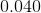expressed in scientific notation?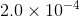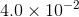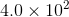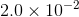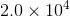Explanation:

The rules of scientific notation are simple. A number is being converted into a format where it's a decimal between 1 and just below 10 (9.9999999...) accompanied by an exponent. The exponent represents how many times to the decimal must move to the left or to the right to become the original number again. Scientific notation does not change the value of the number, but merely provide number in an easier to look at way. If the decimal must move to the right in order to achieve the original number, it will be represented by a positive exponent. If the decimal must move to the left, it will be represented by a negative exponent.

For this example,we first write how this number would be if it were in decimal form (between 1 and just shy of 10).

This would be :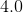Now we must count how many times we had to move the decimal over to achieve this decimal. (Remember where the decimal is in the original number.) We can see that the decimal had to movetimes. With the decimal placed between theand, we see that if we wanted to go back to the original number (), we would have to move the decimal to the left. This means a negative exponent. Therefore, the answer would be:1 3 Next →

### All GED Math Resources